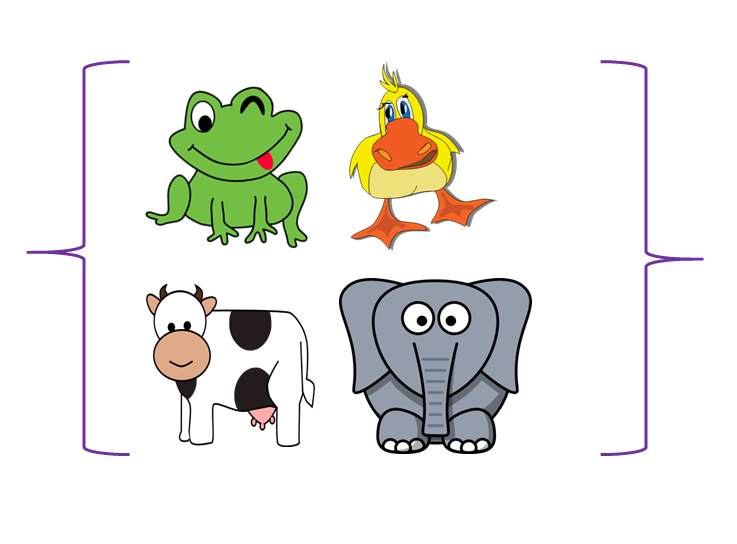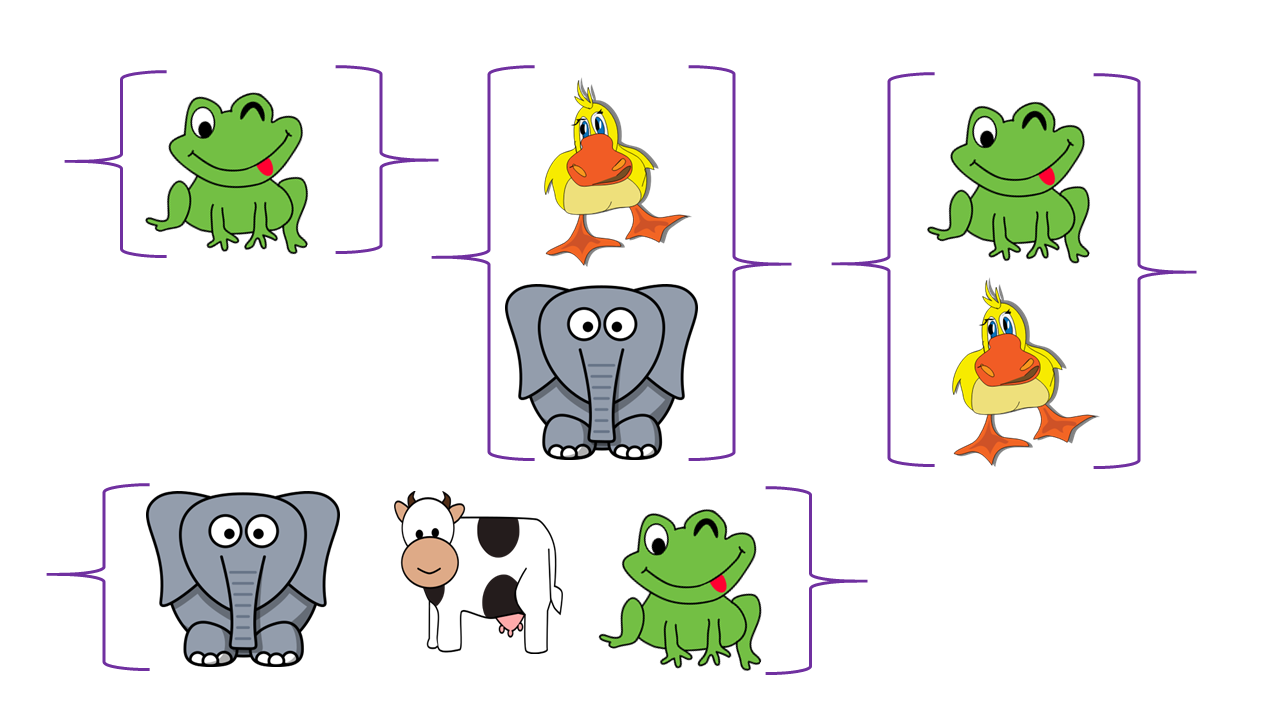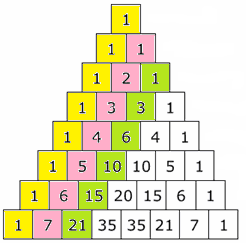# Subsets

A set is a collection of objects. A subset is a set that consists of members of another set. So, a subset is a set that is contained inside another set.then some of its subsets areHowever, it has other subsets as well.

• There's the subset containing no elements. We call this the empty set and write it as $\{\}$ or $\emptyset$.
• Then, there are subsets containing one element. There are four of these: $\{\text{frog}\}, \{\text{duck}\}, \{\text{elephant}\},\{\text{cow}\}$
• Next come the subsets with two elements. There are six:$\{\text{frog},\text{duck}\}, \{\text{frog},\text{elephant}\}, \{\text{frog},\text{cow}\}, \{\text{duck},\text{elephant}\}, \{\text{duck},\text{cow}\}, \{\text{elephant},\text{cow}\}$.
• There are four three element subsets: $\{\text{frog},\text{duck}, \text{elephant}\}, \{\text{frog},\text{elephant},\text{cow}\}, \{\text{frog},\text{cow},\text{duck}\}, \{\text{elephant},\text{cow},\text{duck}\}$
• Finally, the whole set is a subset of itself: $\{\text{frog},\text{duck},\text{elephant},\text{cow}\}$

So, this four element set has $1 + 4 + 6 + 4 + 1 = 16 = 2^4$ subsets.

Let's look at some sets of other sizes and see how many subsets they have.

## The Empty Set

The empty set is the only set that has only one subset. Every set has the set itself and the empty set as subsets, but in this case, they are the same thing.

The only subset of the empty set is the empty set.

The empty set has $1 = 2^0$ subsets.

## A Set with One Element

How many subsets does a set with one element have?

Let's say our one element set is

$\{ \text{frog}\}$

We need to find all the sets that are contained in it.

There are only two choices:

• The emptyset $\emptyset$
• The whole set: $\{\text{frog}\}$

So the set with $1$ element in it has $2$ subsets.

Just, by the way, $2 = 2^1$.

## A Set with Two Elements

How many subsets does a set with two elements have?

Let's say our two element set is

$\{ \text{frog}, \text{duck}\}$

We need to find all the sets that are contained in it.

There are a couple of choices this time, but of course, we have:

• The emptyset $\emptyset$
• The whole set: $\{\text{frog}, \text{duck}\}$

We can also form subsets with one element. There are two possibilities:

• $\{\text{frog}\}$
• $\{\text{duck}\}$

So the set with $2$ elements has $4$ subsets.

Did you notice that $2 = 2^2$?

## A Set with Three Elements

How many subsets does a set with three elements have?

Let's say our three element set is

$\{ \text{frog}, \text{duck}, \text{elephant} \}$

We need to find all the sets that are contained in it.

There are a couple of choices this time, but of course, we have:

• The emptyset $\emptyset$
• The whole set: $\{\text{frog}, \text{duck}, \text{elephant}\}$

We can also form subsets with one element. There are three possibilities:

• $\{\text{frog}\}$
• $\{\text{duck}\}$
• $\{\text{elephant}\}$

Finally, we can form subsets with two elements. There are three of these as well:

• $\{\text{frog}, \text{duck}\}$
• $\{\text{duck}, \text{elephant}\}$
• $\{\text{frog},\text{elephant}\}$

So the set with $3$ elements has $8$ subsets.

Did you notice that $2 = 2^3$?

Now I'm going to leave the work to you.

## Number of Subsets of a Set with Four Elements

How many subsets does a set with four elements have?

Let's say our four element set is

$\{ \text{frog}, \text{duck}, \text{elephant}, \text{cow} \}$

We need to find all the sets that are contained in it. Let's work systematically, and list them by their sizes. I'll name the sizes, you'll do the listing. Be careful, it's really important to get all of them.

• Subsets with 0 elements:
• Subsets with 1 element:
• Subsets with 2 elements:
• Subsets with 3 elements:
• Subsets with 4 elements:

Note: If you got them all, there should be $16$ of them.

## Number of Subsets of a Set with Five Elements

How many subsets does a set with five elements have?

Let's say our five element set is

$\{ \text{frog}, \text{duck}, \text{elephant}, \text{cow}, \text{snail} \}$

We need to find all the sets that are contained in it. Let's work systematically, and list them by their sizes. I'll name the sizes, you'll do the listing. Be careful, it's really important to get all of them.

• Subsets with 0 elements:
• Subsets with 1 element:
• Subsets with 2 elements:
• Subsets with 3 elements:
• Subsets with 4 elements:
• Subsets with 5 elements:

Note: If you got them all, there should be $32$ of them.

## Number of Subsets of a Set with Six Elements

How many subsets does a set with six elements have?

Let's say our six element set is

$\{ \text{frog}, \text{duck}, \text{elephant}, \text{cow}, \text{snail}, \text{echidna} \}$

Hold your horses! There's no need to write them all down. You should have worked out the pattern by now.

How many do you think there should be?

## The Number of Subsets of a Set with $n$ Elements

Did you notice the pattern? Each time we increased the number of elements by $1$, we doubled the number of subsets.

So, the number of subsets of a set with $n$ elements is

$2^n$

A set with $6$ elements has ________________ subsets.

A set with $7$ elements has ________________ subsets.

A set with $8$ elements has ________________ subsets.

## The Numbers of Subsets of Each Size

There's another pattern to spot when we're counting subsets:

• A set with 0 elements has 1 subset.
• A set with 1 element has 1 subset with 0 elements and 1 subset with 1 element.
• A set with 2 elements has 1 subset with 0 elements, 2 subsets with 1 element and 1 subset with 2 elements
• A set with 3 elements has 1 subset with 0 elements, 3 subsets with 1 element, 3 subsets with 2 elements, and 1 subset with 3 elements
• etc.

To me, the green numbers look a lot like the rows of Pascal's triangle:In fact, that's absolutely correct: the rows of Pascal's triangle tell you how many subsets of each size a given set has. For example, the row starting with $1,6$ tells you that a set with 6 elements has

• 1 subset with no elements
• 6 subsets with one element
• 15 subsets with two elements
• 20 subsets with three elements
• 15 subsets with four elements
• 6 subsets with five elements
• 1 subset with six elements

So, the rows of Pascal's triangle let you check that you've written down all the subsets of a set of a particular size, and adding up the numbers in the row lets you know how many subsets a set of that size has.

Since the rth entry in row n of Pascal's triangle is equal to $\begin{pmatrix}n\\r\end{pmatrix}$, we can use this formula to quickly calculate how many subsets of a given size a set has.

For example, a set with $123$ elements has $\begin{pmatrix}123\\ 24\end{pmatrix}$ subsets with 24 elements. We say that this is the number of ways of choosing 24 elements from a set of 123 elements, where the order doesn't matter and repetition is not allowed.

### Description

This chapter series is for Year 10 or higher students, topics include

• Arranging Objects in Lines
• Factorial
• Subsets
• Four colour theorem

and more

### Audience

Year 10 or higher students

### Learning Objectives

These chapters are related to data and in particular "Counting" topics such as Binomial Theorem, Subsets etc

Author: Subject Coach
You must be logged in as Student to ask a Question.